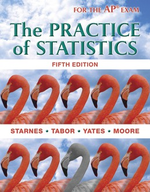×
Get Full Access to The Practice Of Statistics - 5 Edition - Chapter 7.3 - Problem 52
Get Full Access to The Practice Of Statistics - 5 Edition - Chapter 7.3 - Problem 52

×

# Making auto parts Refer to Exercise 50. How many axles would you need to sample if youISBN: 9781464108730 488

## Solution for problem 52 Chapter 7.3

The Practice of Statistics | 5th Edition

• Textbook Solutions
• 2901 Step-by-step solutions solved by professors and subject experts
• Get 24/7 help from StudySoup virtual teaching assistantsThe Practice of Statistics | 5th Edition

4 5 1 256 Reviews
10
1
Problem 52

Making auto parts Refer to Exercise 50. How many axles would you need to sample if you wanted the standard deviation of the sampling distribution of x to be 0.0005 mm? Justify your answer.

Step-by-Step Solution:

Step 1 of 3

Given:Step 2 of 3

Step 3 of 3

## Discover and learn what students are asking

Calculus: Early Transcendental Functions : Integration
?In Exercises 1–6, find the indefinite integral. $$\int\left(5-e^{x}\right) d x$$

Calculus: Early Transcendental Functions : Introduction to Functions of Several Variables
?In Exercises 7-18, find and simplify the function values. $$f(x, y)=2 x+y^{2}$$ (a) $$\frac{f(x+\Delta x, y)-f(x, y)}{\Delta x}$$

Statistics: Informed Decisions Using Data : Tests for Independence and the Homogeneity of Proportions
?Social Well-Being and Obesity The Gallup Organization conducted a survey in 2014 asking individuals questions pertaining to social well-being such as

Statistics: Informed Decisions Using Data : Testing the Significance of the Least-Squares Regression Model
?Kepler’s Law of Planetary Motion The time it takes for a planet to complete its orbit around the sun is called the planet’s sidereal year. Johann Kepl

Unlock Textbook Solution

Enter your email below to unlock your verified solution to:

Making auto parts Refer to Exercise 50. How many axles would you need to sample if you